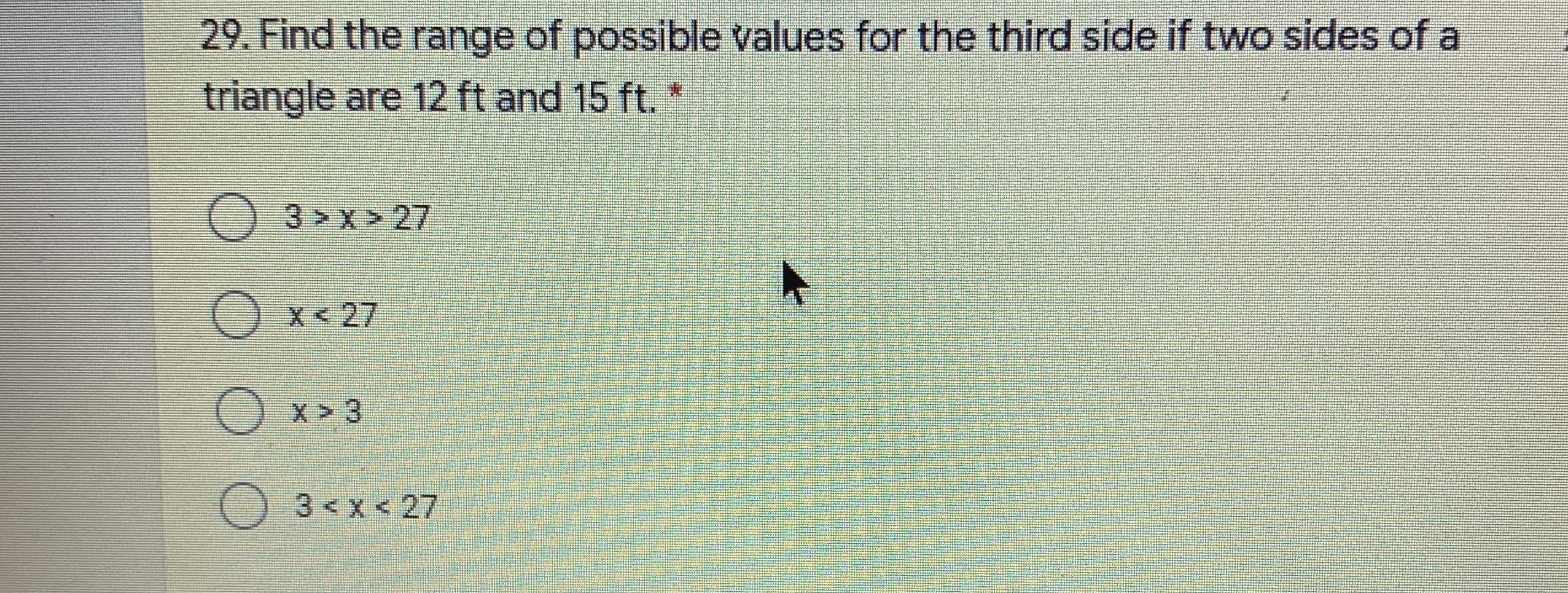### ¿Todavía tienes preguntas de matemáticas?

Pregunte a nuestros tutores expertos
Algebra
Pregunta29. Find the range of possible values for the third side if two sides of a triangle are $$12 ft$$ and $$15 ft$$$$3 > x > 27$$

$$x < 27$$

$$x > 3$$

$$3 < x < 27$$